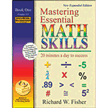#### Fractions - Subtracting Mixed NumbersDo you wonder how to subtract mixed numbers? Do you know how to handle unlike denominators or how to regroup or to borrow with mixed numbers? Take a deep breath and let us take it one step at a time. Click and begin.

I. Subtract Mixed Numerals with Like Denominators
II. Subtract Mixed Numerals with Unlike Denominators

I. Subtract Mixed Numerals with Like Denominators

If you can change a mixed number into an improper fraction and vice versa, you can subtract mixed numbers or mixed fractions that normally require borrowing without doing so.

Steps
1. Change mixed numerals into improper fractions
2. Subtract numerators
3. Do NOT subtract the denominators; the denominator remains the same.
4. Convert improper fraction into a mixed numeral
5. Reduce, if necessary

Example: 7 3/8 – 4 6/8 =

1. Change both fractions into improper fractions --- 59/8 – 38/8

2. Subtract numerators 59 – 38 = 21

3. The denominator remains the same. 8

4. The answer is an improper fraction. Change it into a mixed number.
21/8 = 2 5/8

5. In summary, 7 3/8 – 4 6/8 = 2 5/8

Subtract the same problem by regrouping:
7 3/8 - 4 6/8 =
1. Regroup, if necessary.
It is not possible to subtract 6 from 3. So, borrow one from 7

1B. Add that one to 3/8 as follows 3/8 + 8/8 (remember any number over itself equals one)
the problem now reads 6 11/8 - 4 6/8 =

2. Subtract numerators: 11 – 6 = 5

3. The denominator remains the same: 8

4. Subtract the whole numbers: 6 - 4 = 2

5. In summary: 7 3/8 - 4 6/8 = 6 11/8 - 4 6/8 = 2 5/8

Which method do you prefer?

II. Subtract Mixed Numerals with Unlike Denominators
(Don’t you run. Come back. You can do this. Just Believe!)

Remember: Denominators must be the same in order to subtract or add fractions

Example: 9 5/7 minus 3 4/6
Good Math Detectives will notice the denominators are not the same.

Steps
1. Find the least common multiple between the two denominators. Most commonly referred to as the least common denominator (LCD)
2. Find the equivalent fraction for each fraction using the LCD. (As a result, the denominators are the same.)
3. Subtract the numerators (The denominators are NOT subtracted.)
4. The denominator remains the same.
5. Subtract the whole numbers.
6. Reduce, if necessary

1. Find the least common denominator (LCD) : To find the LCD, use prime factorization. (refer to GCF and LCM by Prime Factorization article) Another way is to count by 6’s and count by 7’s. The first number they have in common is the LCD. One more way is to simply multiply the denominators. If you multiply the denominators, the result is not necessarily the lowest denominator. This is okay; it just means you must remember to reduce. Right now, let us use prime factorization method. The prime factorization for 6 is 2 * 3. The number 7 is a prime number. The LCD for 42 = 2*3*7 . It just so happens, if you were to multiply the denominators, the answer is still 42. (Please refer to the Prime Factorization article for a more detailed explanation.)

2. Find the equivalent fraction for each fraction using the LCD.
Since the new denominator is 42. New numerators are needed. 4/6 = ?/42
Ask yourself , the denominator,6, times what number equals 42. It is 7. Since, the denominator was multiplied by 7, the numerator must be multiplied by 7. Thus the equivalent fraction for 4/6 using 42 as the denominator is 28/42. . Next, 5/7 = ?/42. Ask yourself , the denominator,7, times what number equals 42. It is 6. Since, the denominator was multiplied by 6, the numerator must be multiplied by 6. Thus, the equivalent fraction for 5/7 using 42 as the denominator is 30/42.
Now, the problem reads: 9 30/42 - 3 28/42 =

3. Subtract the numerators: 30 - 28 = 2

4. The denominator remains the same: 42

5. Subtract the whole numbers: 9 – 3 = 6
Therefore: 9 30/42 - 3 28/42 = 6 2/42

5. Reducing is necessary because 2 and 42 have at least one common factor.
2/42 = 1/21 (If necessary, refer to article on reducing at the end of this article.)
Therefore: 9 5/7 minus 3 4/6 = 9 30/42 - 3 28/42 = 2/42 = 6 1/21

It's time to practice! You may purchase a book with short concise practice lessons below from
Christianbook.com HomeMastering Essential Math Skills, Revised Edition: Book One Produce motivated and confident math students with this innovative, easy-to-use system of teaching basic math! Each daily lesson contains five key components: two speed drills, review exercises, Helpful Hints, new material and a daily word problem. Lessons are not rigid however; teachers have the flexibility to modify lesson plans while structure and a basic framework are still provided. Eight chapters cover whole numbers, fractions, decimals, percentages, integers, geometry, charts, graphs and problem solving. Help your students really master the essential math skills they'll need throughout their lives with enthusiasm and consistency. Answer key and final review included. 142 pages, softcover. For Grades 4-5. Book 1.

Converting Improper Fractions and Mixed Numbers
GCF and LCM by Prime Factorization
Reducing FractionsRelated Articles
Editor's Picks Articles
Top Ten Articles
Previous Features
Site Map